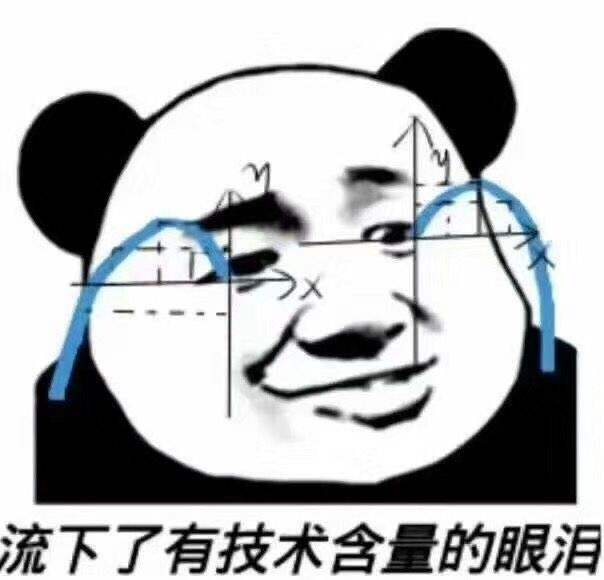## 导语

OK，言归正传，今天闲来无事，我翻出了女儿去年玩过的小游戏，她妈妈给她买的这类智力小游戏有不少，很偶尔会瞧见她们娘俩一起玩，估计是不太感兴趣。今天这款游戏，我小时候没见过，是一个新的小游戏，我就起一个应景的名字，《兔兔大战小狐狸》，今年是兔年，兔子要逃脱小狐狸的围追堵截，逃到安全的小土坑里，躲起来。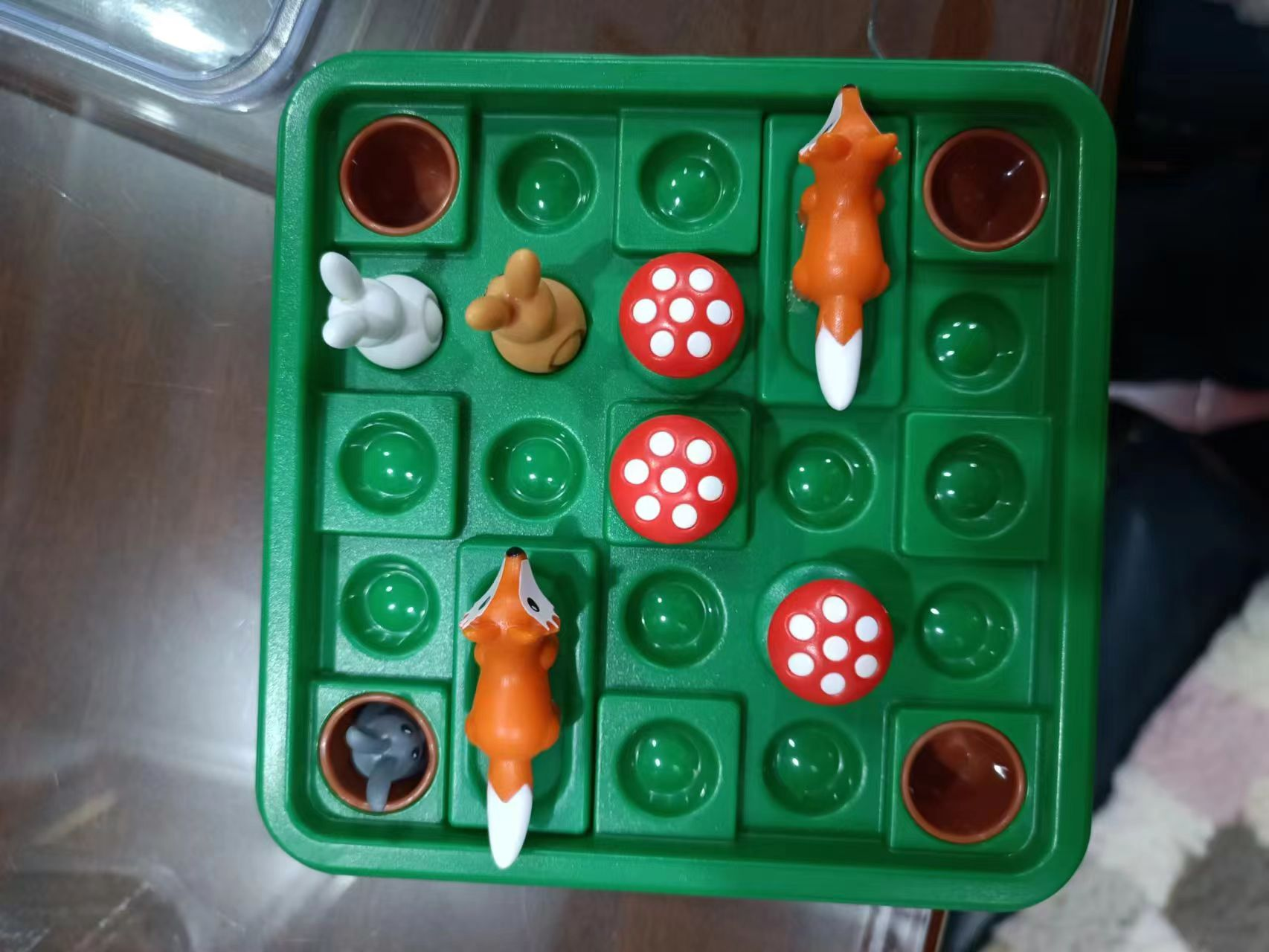## 游戏介绍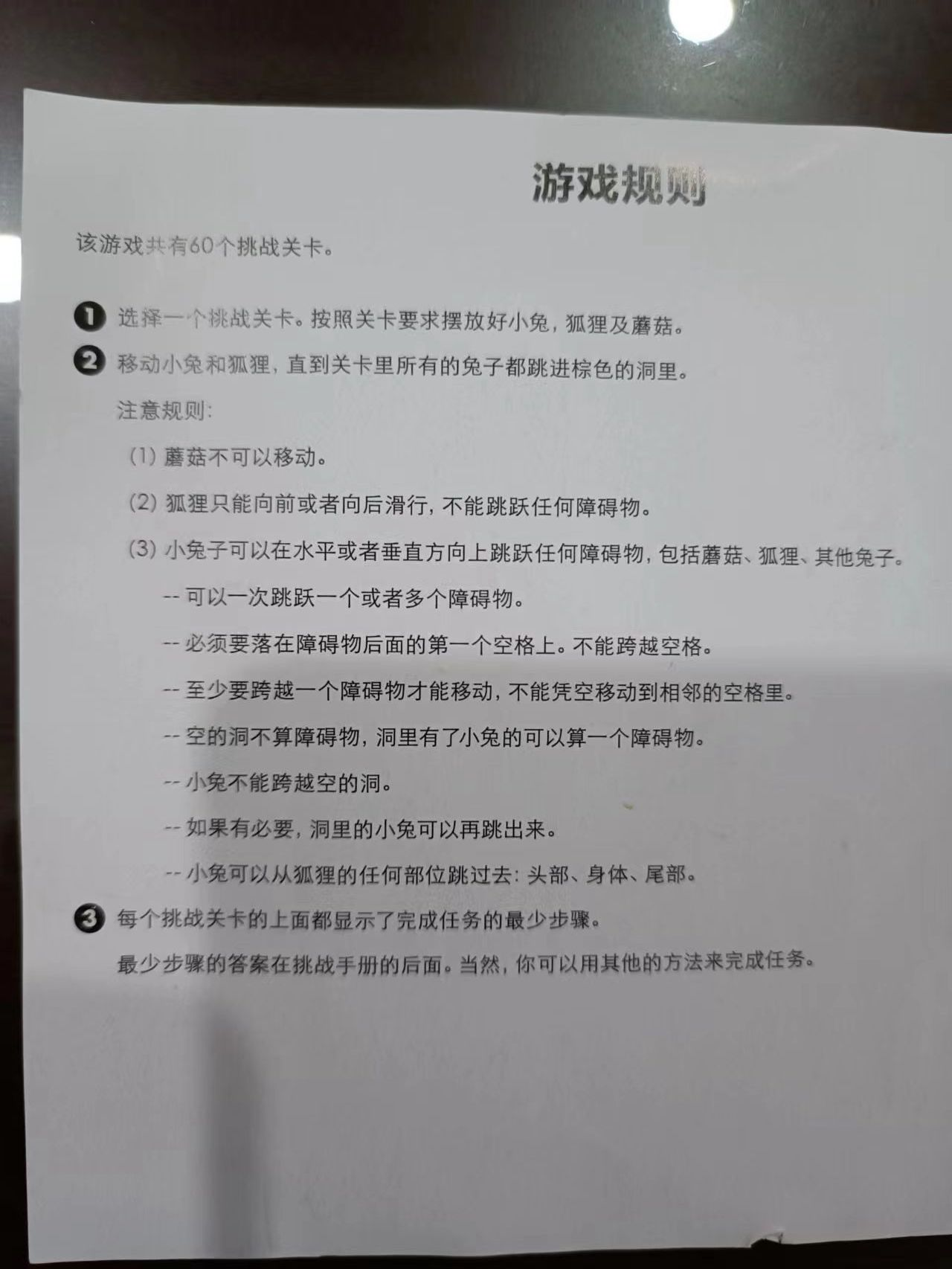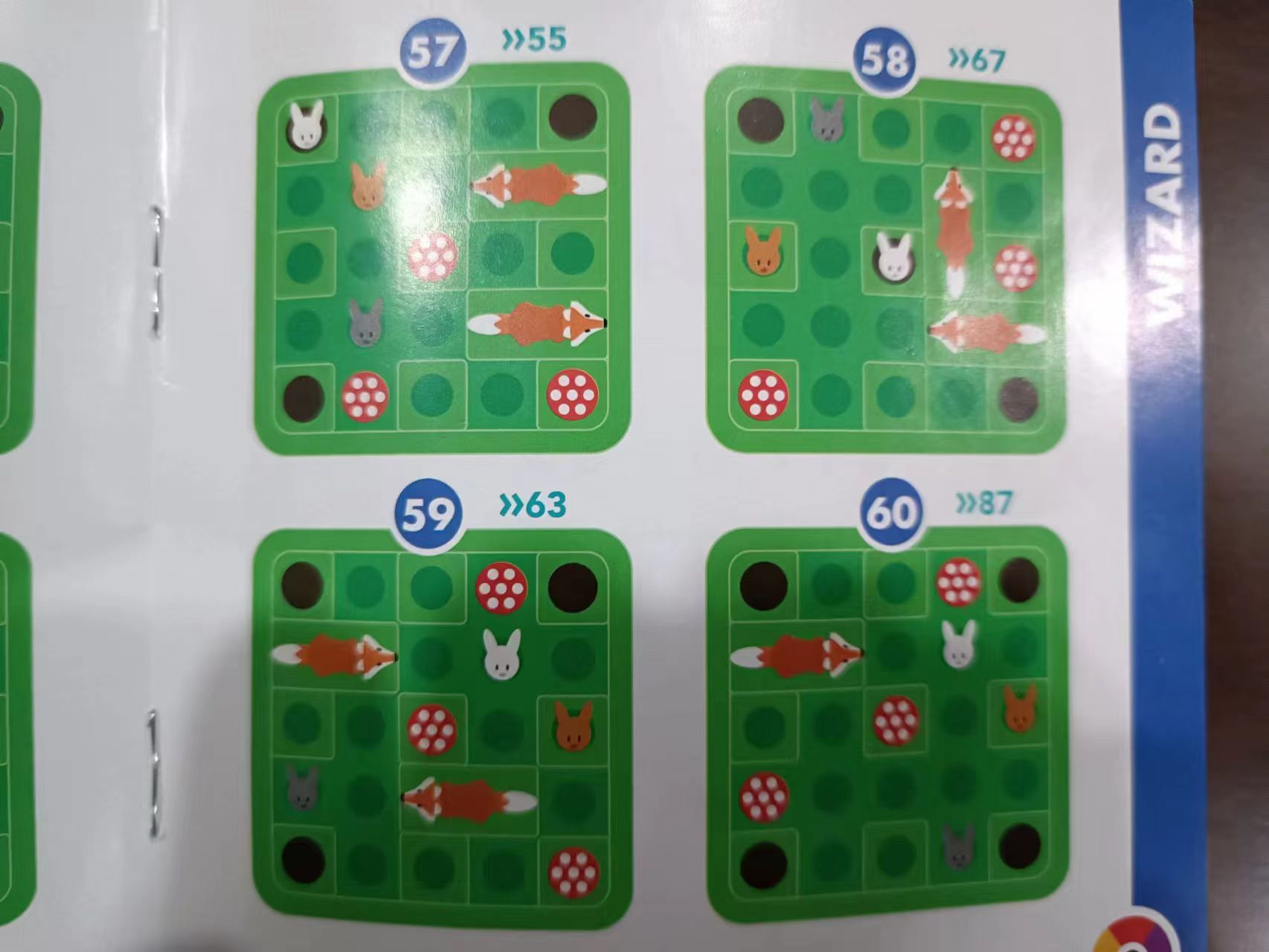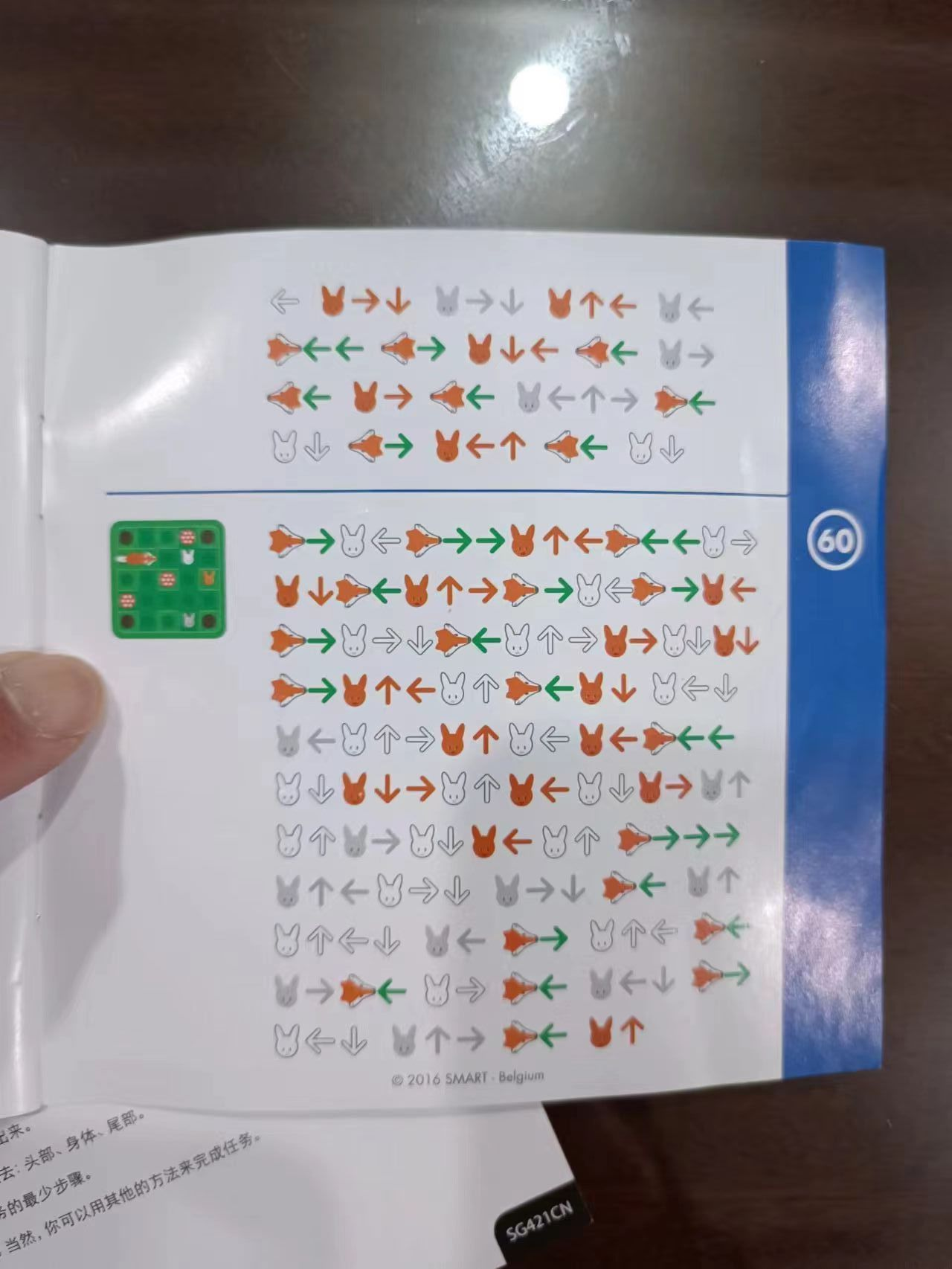## 求解过程

``````"...X."
"FF.R."
"..X.R"
"X...."
"...R."
``````

OK，那么我就来试下BFS搜索，大概的逻辑步骤如下：

``````1. 建立一个队列，将游戏初始棋盘局面放到队列中；
2. 一一取出当前局面，评估可能变为的下一个局面；
3. 评估当前局面可能可以行动的小兔兔、小狐狸坐标；
4. 一一取出小兔兔、小狐狸，评估她们可能的移动到的位置；
5. 通过改变小兔兔、小狐狸位置，得到下一个局面；
6. 将2～5变化的到的局面插入队列（下一轮）；
7. 遍历完上一轮队列，步骤+1；
8. 把下一轮队列变为需要遍历的当前队列，重复2～7步骤；
``````

``````int smartGame(vector<string> board) {
int n = board.size();

// 判断游戏是否结束
set<vector<int>> finish_pos = {{0, 0}, {0, n-1}, {n-1, 0}, {n-1, n-1}, {n/2, n/2}};
function<bool(vector<vector<int>>)> isFinished = [&](vector<vector<int>> ani_pos) -> bool {
int n = ani_pos.size();

for (int i = 0; i < n; ++i) {
if (ani_pos[i] != -1) continue;
vector<int> pos = {ani_pos[i], ani_pos[i]};
if (finish_pos.find(pos) == finish_pos.end())
return false;
}

return true;
};

// 分析棋盘局面，哪些可能移动的小兔兔、小狐狸所在位置
function<void(vector<string>, vector<vector<int>>&)> analyzeBoard = [&](vector<string> board, vector<vector<int>>& ani_pos) {
for (int i = 0; i < n; ++i) {
for (int j = 0; j < n; ++j) {
if (board[i][j] == 'X') {
continue;
} else if (board[i][j] == '.') {
continue;
} else if (board[i][j] == 'F') {
if ((i == 0 || board[i - 1][j] != 'F') && (i < n - 1 && board[i + 1][j] == 'F')) {
// vertical fox
ani_pos.push_back({i, j, 1});
} else if ((j == 0 || board[i][j - 1] != 'F') && (j < n - 1 && board[i][j + 1] == 'F')) {
// horizontal fox
ani_pos.push_back({i, j, 2});
}
} else if (board[i][j] == 'R') {
ani_pos.push_back({i, j, -1});
}
}
}
};

// 棋盘局面上某个小兔兔、小狐狸，做出某个移动后，返回新的局面
function<vector<string>(vector<string>, vector<int>, vector<int>)> applyBoard = [&] (vector<string> board, vector<int> pos, vector<int> move) -> vector<string> {
if (pos == -1) {
board[pos][pos] = '.';
board[pos+move][pos+move] = 'R';
} else {
if (pos == 1) {
board[pos][pos] = '.';
board[pos+1][pos] = '.';

board[pos+move][pos+move] = 'F';
board[pos+move+1][pos+move] = 'F';
} else if (pos == 2) {
board[pos][pos] = '.';
board[pos][pos+1] = '.';

board[pos+move][pos+move] = 'F';
board[pos+move][pos+move+1] = 'F';
}
}

return board;
};

// 分析棋盘局面，可能移动的小兔兔、小狐狸，能做出哪些移动
function<vector<vector<int>>(vector<string>, vector<int>)> nextMoves = [&] (vector<string> board, vector<int> pos) -> vector<vector<int>> {
vector<vector<int>> moves;

if (pos == -1) {
// up
vector<int> t = pos;
while (t >= 0 && board[t][t] != '.') t -= 1;
if (t >= 0 && board[t][t] == '.' && t < pos-1) {
moves.push_back({t-pos, 0});
}
// down
t = pos;
while (t < n && board[t][t] != '.') t += 1;
if (t < n && board[t][t] == '.' && t > pos+1) {
moves.push_back({t-pos,0});
}
// left
t = pos;
while (t >= 0 && board[t][t] != '.') t -= 1;
if (t >= 0 && board[t][t] == '.' && t < pos-1) {
moves.push_back({0, t-pos});
}
// right
t = pos;
while (t < n && board[t][t] != '.') t += 1;
if (t < n && board[t][t] == '.' && t > pos+1) {
moves.push_back({0, t-pos});
}
} else if (pos.size() == 3) {
if (pos == 1) {
// up
if (pos > 0 && board[pos-1][pos] == '.') {
moves.push_back({-1, 0});
}
// down
if (pos < n-2 && board[pos+2][pos] == '.') {
moves.push_back({1, 0});
}
} else if (pos == 2) {
// left
if (pos > 0 && board[pos][pos-1] == '.') {
moves.push_back({0, -1});
}
// right
if (pos < n-2 && board[pos][pos+2] == '.') {
moves.push_back({0, 1});
}
}
}

return moves;
};

// 棋盘局面的签名，用作去重
function<string(vector<string>)> signature = [&](vector<string> board) -> string {
string r;
for (auto l : board) {
r += l;
}
return r;
};

// 1. 初始棋盘局面，入到队列
queue<vector<string>> q;
unordered_set<string> seen;
q.push(board);
seen.insert(signature(board));

int ans = 0;

while (!q.empty()) {
int size = q.size();
while (size --) {
// 2. 一一取出当前局面，评估可能变为的下一个局面；
vector<string> t = q.front();
q.pop();
vector<vector<int>> ani_pos;

// 3. 评估当前局面可能可以行动的小兔兔、小狐狸坐标；
analyzeBoard(t, ani_pos);

if (isFinished(ani_pos)) {
return ans;
}

// 4. 一一取出小兔兔、小狐狸，评估她们可能的移动到的位置；
for (auto pos: ani_pos) {
vector<vector<int>> moves = nextMoves(t, pos);
for (int i = 0; i < moves.size(); ++i) {
vector<int> move = moves[i];
// 5. 通过改变小兔兔、小狐狸位置，得到下一个局面；
vector<string> nxtBoard = applyBoard(t, pos, move);
string sig = signature(nxtBoard);
if (seen.find(sig) == seen.end()) {
// 6. 将2～5变化的到的局面插入队列（下一轮）；
q.push(nxtBoard);
seen.insert(sig);
}
}
}
}

// 7. 遍历完上一轮队列，步骤+1；
// 8. 把下一轮队列变为需要遍历的当前队列，重复2～7步骤；
ans++;
}

return -1;
}
``````

## 图像手绘风格• 黑白灰色
• 边界线条较重
• 相邻的像素，如果是相同或者相近色彩趋于白色
• 略有光源效果

## 梯度的重构

``````import numpy as np
from PIL import Image

a = np.asarray(Image.open('xxx.jpeg').convert('L')).astype('float')

depth = 10.
``````

``````a = np.asarray(Image.open('xxx.jpeg').convert('L')).astype('float')
``````

``````depth = 10.
``````

## 光源效果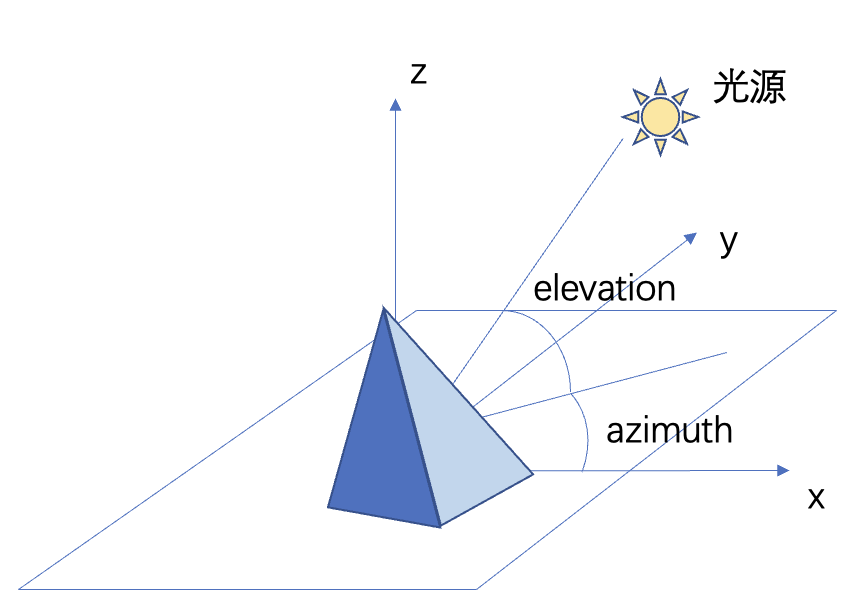• 设计一个位于图像斜上方的虚拟光源
• 光源相对于图像的俯视角为Elevation，方位角为Azimuth
• 建立光源对各个点梯度值的影响函数
• 运算各个点的新像素值

``````vec_el = np.pi/2.2                  # 光源的俯视角度，弧度值
vec_az = np.pi/4.                   # 光源的方位角度，弧度值
dx = np.cos(vec_el)*np.cos(vec_az)  # 光源对x轴的影响
dy = np.cos(vec_el)*np.sin(vec_az)  # 光源对y轴的影响
dz = np.sin(vec_el)                 # 光源对z轴的影响

uni_z = 1./A
b = 255*(dx*uni_x+dy*uni_y+dz*uni_z)
``````

``````b = b.clip(0, 255)
im = Image.fromarray(b.astype('uint8'))
im.save('xxxHD.jpeg')
``````

## 导语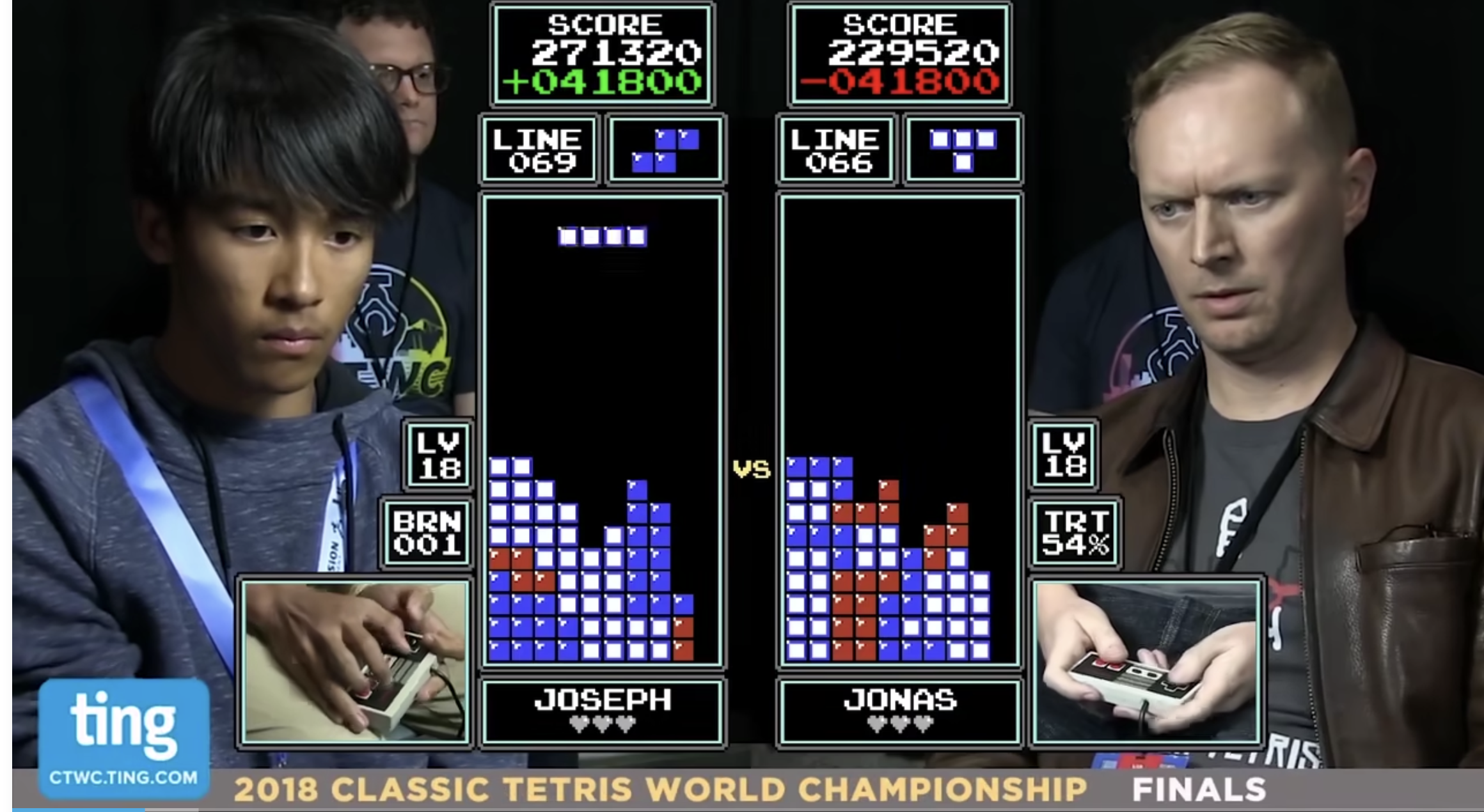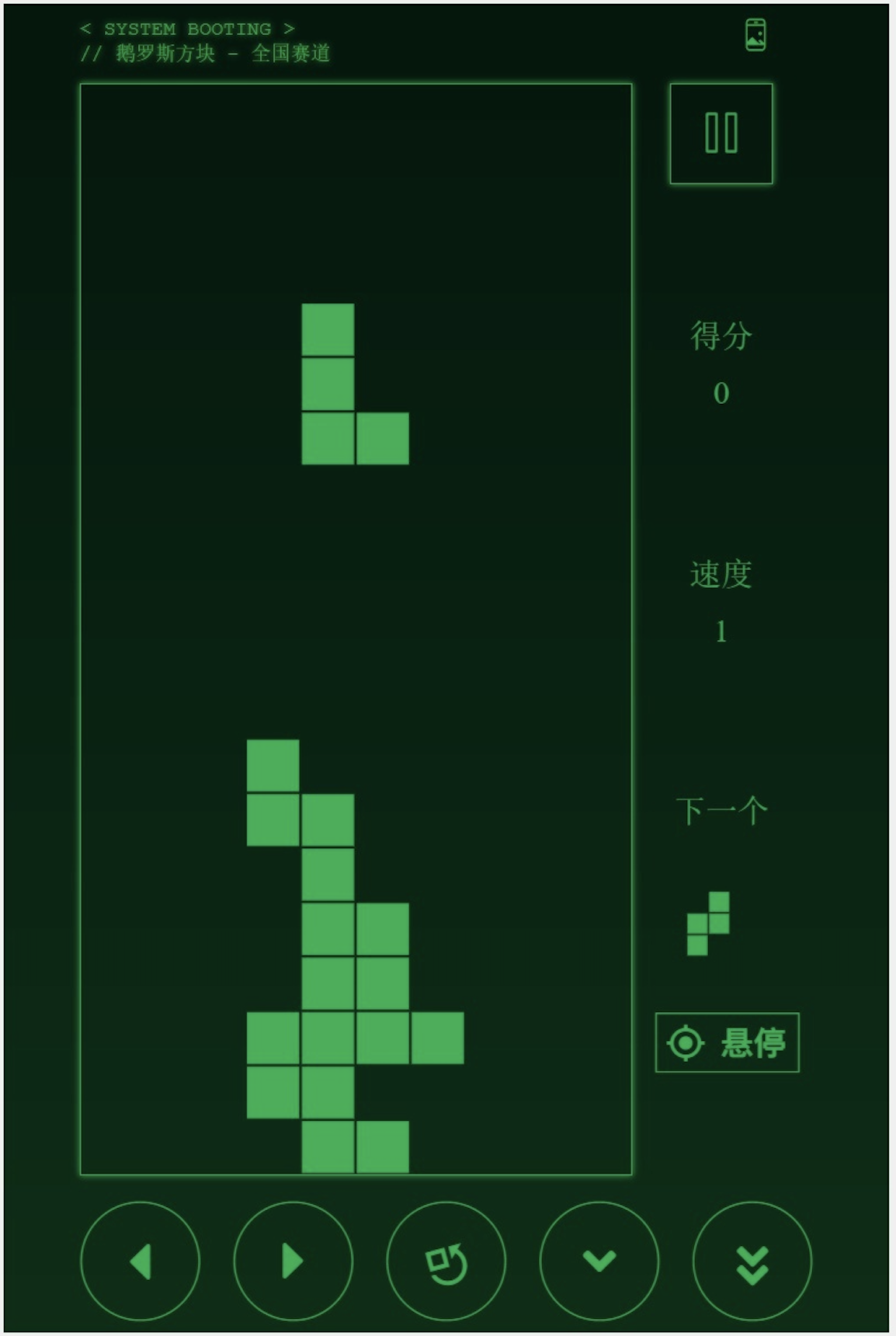## 如何活着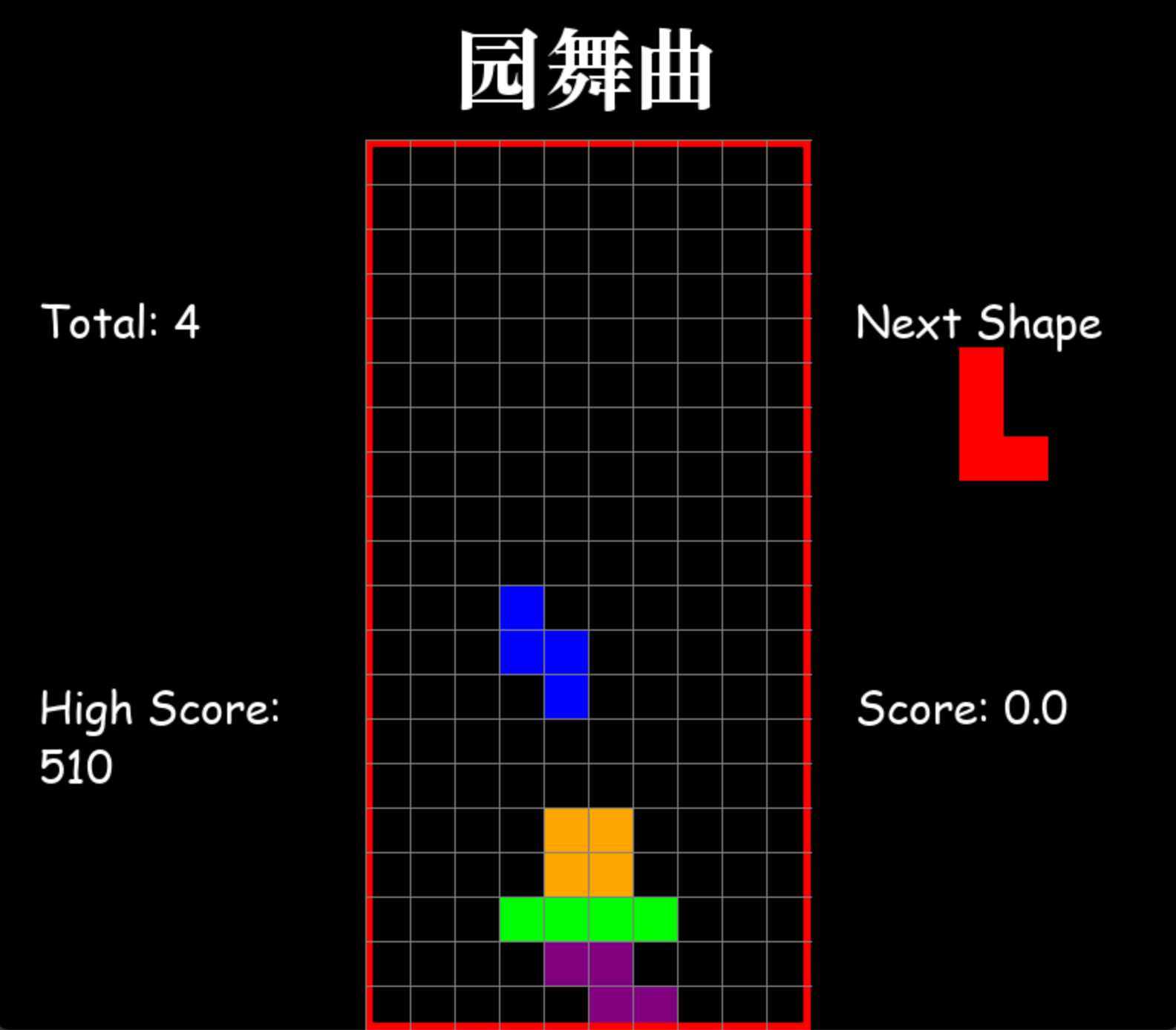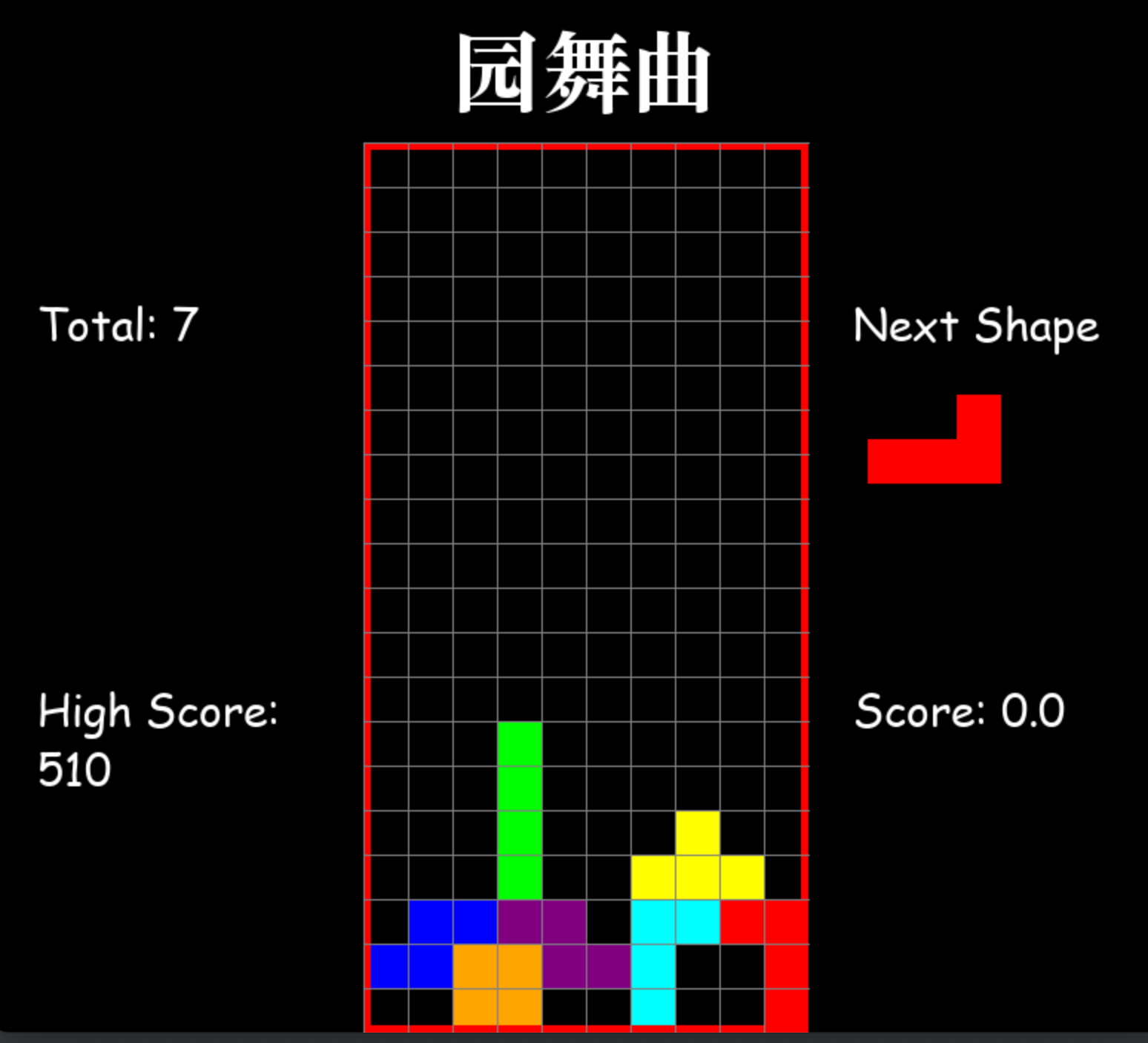``````BrickShapes = [
# I
[[[-2, 0], [-1, 0], [0, 0], [1, 0]],
[[0, -1], [0, 0], [0, 1], [0, 2]]],

# L
[[[-2, 0], [-1, 0], [0, 0], [0, 1]],
[[0, 0], [0, 1], [0, 2], [1, 0]],
[[0, -1], [0, 0], [1, 0], [2, 0]],
[[-1, 0], [0, -2], [0, -1], [0, 0]]],

# J
[[[-2, 0], [-1, 0], [0, -1], [0, 0]],
[[-1, 0], [0, 0], [0, 1], [0, 2]],
[[0, 0], [0, 1], [1, 0], [2, 0]],
[[0, -2], [0, -1], [0, 0], [1, 0]]],

# T
[[[0, -1], [0, 0], [0, 1], [1, 0]],
[[-1, 0], [0, -1], [0, 0], [1, 0]],
[[-1, 0], [0, -1], [0, 0], [0, 1]],
[[-1, 0], [0, 0], [0, 1], [1, 0]]],

# O
[[[-1, 0], [-1, 1], [0, 0], [0, 1]]],

# S
[[[-1, 0], [-1, 1], [0, -1], [0, 0]],
[[-1, -1], [0, -1], [0, 0], [1, 0]]],

# Z
[[[-1, -1], [-1, 0], [0, 0], [0, 1]],
[[-1, 0], [0, -1], [0, 0], [1, -1]]]
]``````

``````    # I, two rotations
[[[-2, 0], [-1, 0], [0, 0], [1, 0]],
[[0, -1], [0, 0], [0, 1], [0, 2]]],
``````

El-Tetris算法是传统AI人工智能，不要因为人工智能，就觉得很复杂，其实不论传统的AI算法还是目前深度学习的人工算法，一般都不会很复杂，深度学习AI算法不在此文讨论范围。基本上传统AI算法就是列举所有的可能性，通过计算预先建模的公式，评估最优秀的一个局面，做出选择，一直循环往复，直到游戏结束。我们看下El-Tetris算法的公式：
f(x) = b0x0+b1x1+b2x2...bnxn

``````while the game is not over:
examine piece given
find the best move possible (an rotation and a position)
play piece
repeat
``````

• Landing Height: The height where the piece is put (= the height of the column + (the height of the piece / 2)).
• Rows eliminated: The number of rows eliminated.
• Row Transitions: The total number of row transitions. A row transition occurs when an empty cell is adjacent to a filled cell on the same row and vice versa.
• Column Transitions: The total number of column transitions. A column transition occurs when an empty cell is adjacent to a filled cell on the same column and vice versa.
• Number of Holes: A hole is an empty cell that has at least one filled cell above it in the same column.
• Well Sums: A well is a succession of empty cells such that their left cells and right cells are both filled.

Landing Height和Rows eliminated比较好理解，其他对着下图解释下：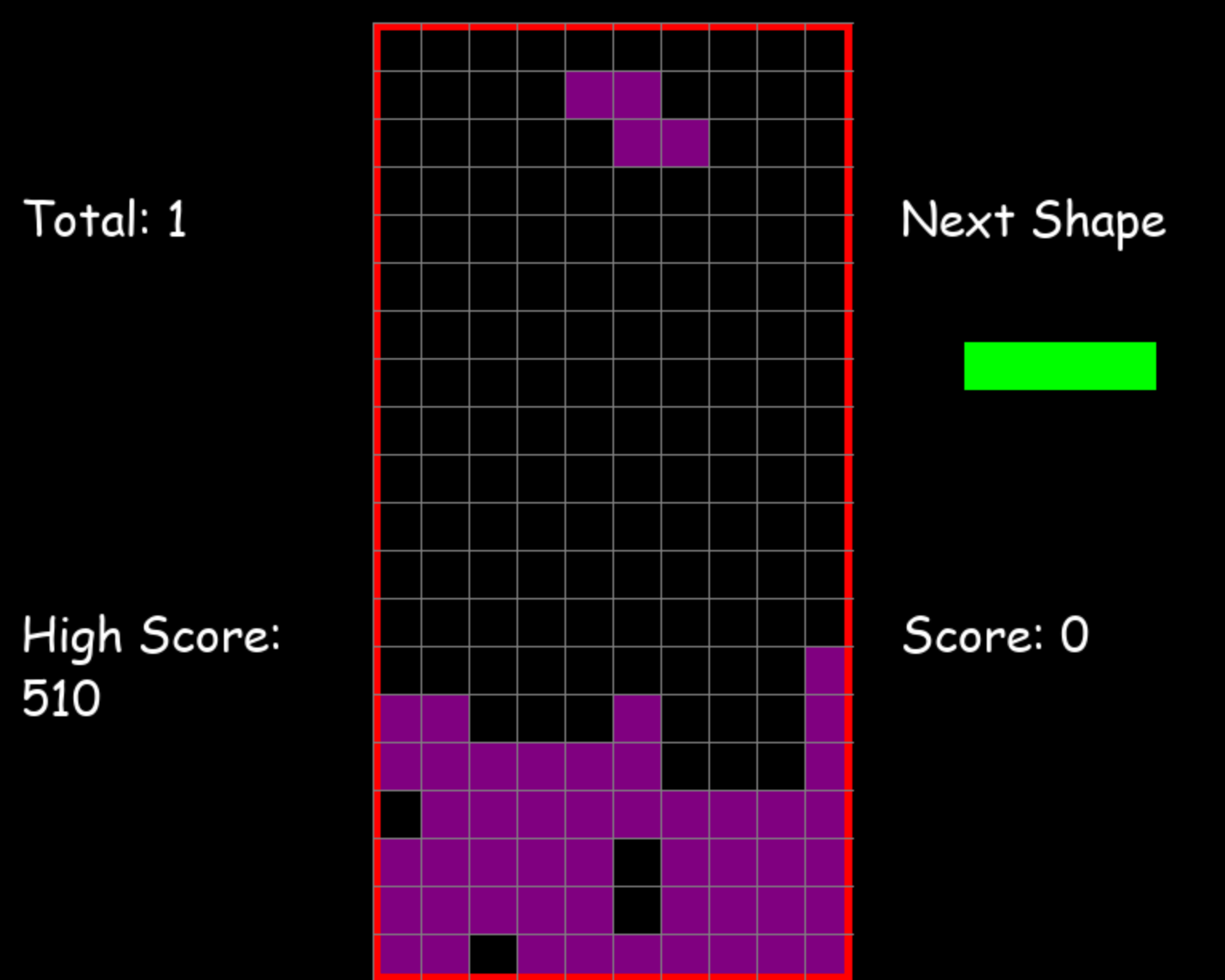Row Transitions总数是16，最底部一行为2，依次往上2、2、2、2、4、2，注意我这里处理为左右边界外是被占据的，所以倒数第四行靠最左边有个空，算存在一次row transition，另外空行我没算（我猜测也可以计算row transition，不影响最后结果）。
Column Transitions总数是26，如果理解了Row Transitions，这个计算方法是一样的。
Number of Holes总数是4，看下上图，应该知道什么叫“洞”，被封闭了的空单元格。
Well Sums总数是5，如果左右两边单元格被占，顶上又没有被封闭，可以算作一个“井”格子，第一列一个单元格，左边界外算占据，右边也被占据，所以也算作“井”格子，深度为1，第三列就一个单元格，井深度为1，第六列有2个空单元格，井深度和为1+1+1+2。这个比较难理解，多看几遍。

Landing Height = -4.500158825082766
Rows eliminated = 3.4181268101392694
Row Transitions = -3.2178882868487753
Column Transitions = -9.348695305445199
Number of Holes = -7.899265427351652
Well Sums = -3.3855972247263626

``````def find_best_play(current_piece, grid):
if current_piece.y < 0:
False, 0, 0

rotations = len(current_piece.shape)
x_moves = [-5, -4, -3, -2, -1, 0, 1, 2, 3, 4, 5]
rotation = 0
x_move = 0
best_play_score = float("-inf")
for i in range(rotations):
for j in x_moves:
candidate_piece = copy.deepcopy(current_piece)
candidate_grid = copy.deepcopy(grid)
candidate_piece.rotation += i
candidate_piece.x += j

if not (valid_space(candidate_piece, candidate_grid)):
continue

valid, score = evaluate(candidate_piece, candidate_grid)
if valid and score > best_play_score:
best_play_score = score
rotation = i
x_move = j

return True, rotation, x_move

def evaluate(current_piece, grid):
while True:
current_piece.y += 1
if not (valid_space(current_piece, grid)) and current_piece.y > 0:
current_piece.y -= 1
break

shape_pos = convert_shape_format(current_piece)

for i in range(len(shape_pos)):
x, y = shape_pos[i]
if y > -1:
grid[y][x] = current_piece.color

heights_points = (20 - current_piece.y + current_piece.height[current_piece.rotation % len(current_piece.height)]) * float(-4.500158825082766)
rows_removed = get_rows_removed(grid) * float(6.4181268101392694)
rows_transitions = get_row_transitions(grid) * float(-3.2178882868487753)
cols_transitions = get_col_transitions(grid) * float(-9.348695305445199)
num_of_holes = get_num_of_holes(grid) * float(-14.899265427351652)
wells = get_well_sums(grid) * float(-6.3855972247263626)

return True, heights_points + rows_removed + rows_transitions + cols_transitions + num_of_holes + wells
``````

find_best_play列举所有可能的操作，evaluate就是评估局面的分数，其中几个计算特征值的函数：

``````def get_row_transitions(grid):
rows = len(grid)
cols = len(grid)
transitions = 0
last_pos = True
for i in range(rows - 1, -1, -1):
if is_empty_row(grid[i]):
continue

for j in range(cols):
if (grid[i][j] in brick.SHAPES_COLORS) != last_pos:
transitions += 1

last_pos = (grid[i][j] in brick.SHAPES_COLORS)

if not last_pos:
transitions += 1

last_pos = True

return transitions

def get_col_transitions(grid):
rows = len(grid)
cols = len(grid)
transitions = 0
last_pos = True
for j in range(cols):
for i in range(rows - 1, -1, -1):
if (grid[i][j] in brick.SHAPES_COLORS) != last_pos:
transitions += 1

last_pos = (grid[i][j] in brick.SHAPES_COLORS)

if not last_pos:
transitions += 1

last_pos = True

return transitions

def get_num_of_holes(grid):
rows = len(grid)
cols = len(grid)
holes = 0
previous_row_holes = [0 for _ in range(cols)]
for i in range(rows)[1:]:
for j in range(cols):
if grid[i][j] == (0, 0, 0) and (grid[i-1][j] != (0, 0, 0) or previous_row_holes[j] == 1):
holes += 1
previous_row_holes[j] = 1

return holes

def get_well_sums(grid):
rows = len(grid)
cols = len(grid)
well_sums = 0

# Check for well cells in the "inner columns" of the board.
# "Inner columns" are the columns that aren't touching the edge of the board.
for j in range(cols-1)[1:]:
for i in range(rows - 1, -1, -1):
if grid[i][j] == (0, 0, 0) and grid[i][j-1] != (0, 0, 0) and grid[i][j+1] != (0, 0, 0):
well_sums += 1

k = i+1
while k < rows:
if grid[k][j] == (0, 0, 0):
well_sums += 1
else:
break
k += 1

# Check for well cells in the leftmost column of the board.
for i in range(rows - 1, -1, -1):
if grid[i] == (0, 0, 0) and grid[i] != (0, 0, 0):
well_sums += 1

k = i + 1
while k < rows:
if grid[k] == (0, 0, 0):
well_sums += 1
else:
break
k += 1

# Check for well cells in the rightmost column of the board.
for i in range(rows - 1, -1, -1):
if grid[i][cols-1] == (0, 0, 0) and grid[i][cols-2] != (0, 0, 0):
well_sums += 1

k = i + 1
while k < rows:
if grid[k][cols-1] == (0, 0, 0):
well_sums += 1
else:
break
k += 1

return well_sums
``````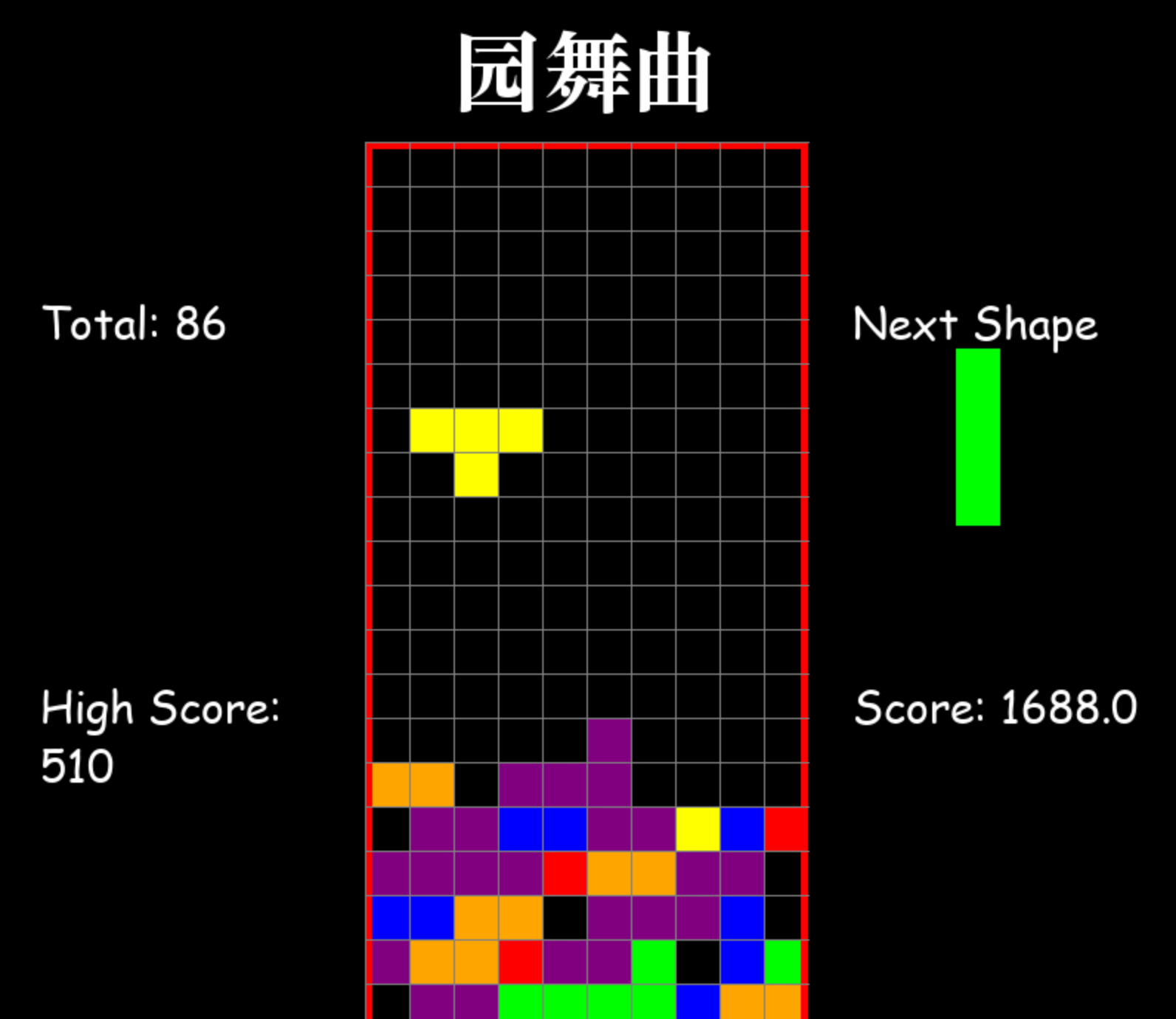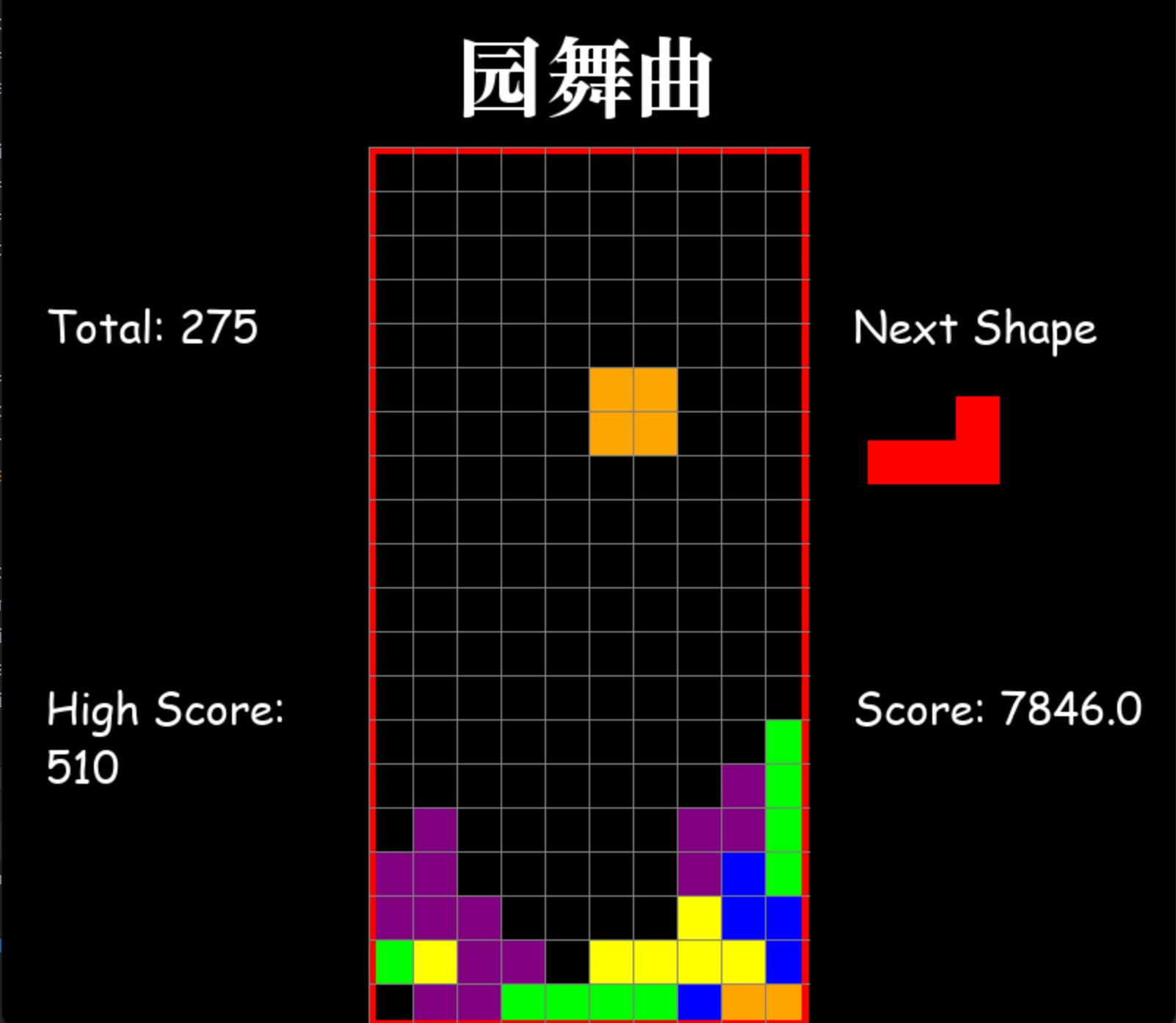``````def getShapeIndex(self):
weight = self.rand_number % 29
shape_index = 0

if 0 <= weight <= 1:
shape_index = 0
elif 1 < weight <= 4:
shape_index = 1
elif 4 < weight <= 7:
shape_index = 2
elif 7 < weight <= 11:
shape_index = 3
elif 11 < weight <= 16:
shape_index = 4
elif 16 < weight <= 22:
shape_index = 5
else:
shape_index = 6

return shape_index
``````

## 追求“财富”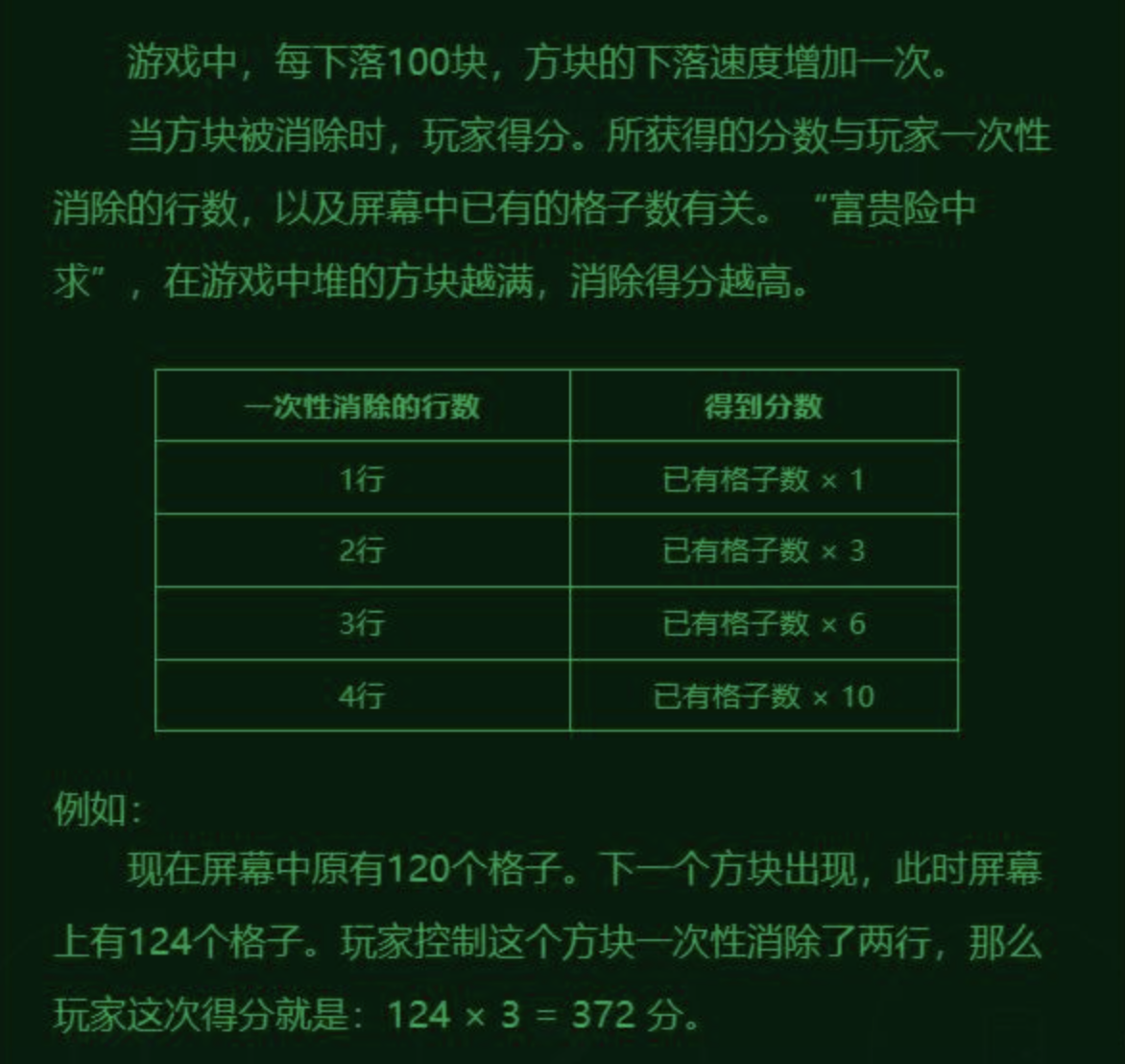``````evaluation = board_evaluator.Evaluate(k) - v.score * self.score_weight
``````

``````PARAMS = [
-1,
3.2178882868487753,
9.348695305445199,
7.899265427351652
# 3.3855972247263626
]

def Evaluate(board):
return PARAMS * board.getCount() + PARAMS * board.getRowTransition() + \
PARAMS * board.getColTransition() + PARAMS * board.getNumberOfHoles()
``````## 导语## 摸索

``````bosses = [
{
"Name": "小壶守卫",
"Model": "Boss_ball",
"Hp": 396,
"Skill1_harm": 63,
"Skill2_harm": 71,
"Award_hp": 133,
"Award_mp": 1695,
"New_star": 0
},
{
"Name": "苇名二心",
"Model": "Boss_sword",
"Hp": 532,
"Skill1_harm": 59,
"Skill2_harm": 89,
"Award_hp": 157,
"Award_mp": 1678,
"New_star": 0
},
{
"Name": "古达",
"Model": "Boss_guda",
"Hp": 643,
"Skill1_harm": 57,
"Skill2_harm": 97,
"Award_hp": 142,
"Award_mp": 1362,
"New_star": 1
},
{
"Name": "中壶守卫",
"Model": "Boss_ball",
"Hp": 668,
"Skill1_harm": 59,
"Skill2_harm": 67,
"Award_hp": 178,
"Award_mp": 1458,
"New_star": 0
},
{
"Name": "苇名三心",
"Model": "Boss_sword",
"Hp": 687,
"Skill1_harm": 31,
"Skill2_harm": 101,
"Award_hp": 212,
"Award_mp": 2659,
"New_star": 0
},
{
"Name": "黑古达",
"Model": "Boss_guda",
"Hp": 852,
"Skill1_harm": 77,
"Skill2_harm": 91,
"Award_hp": 132,
"Award_mp": 4157,
"New_star": 2
},
{
"Name": "大壶守卫",
"Model": "Boss_ball",
"Hp": 1502,
"Skill1_harm": 71,
"Skill2_harm": 105,
"Award_hp": 154,
"Award_mp": 3089,
"New_star": 0
},
{
"Name": "苇名零心",
"Model": "Boss_sword",
"Hp": 1611,
"Skill1_harm": 97,
"Skill2_harm": 127,
"Award_hp": 189,
"Award_mp": 6493,
"New_star": 0
},
{
"Name": "超黑古达",
"Model": "Boss_guda",
"Hp": 2988,
"Skill1_harm": 101,
"Skill2_harm": 153,
"Award_hp": 0,
"Award_mp": 0,
"New_star": 3
}
]``````

``````hero = {
"Hp": 520,
"Mp": 1806,
"Power": {
"Cost_mp": 233,
"Attack_increase": [
{
"skill_i": 1,
"skill_j": 1,
"skill_k": 1,
"skill_l": 1,
"skill_u": 1
},
{
"skill_i": 6,
"skill_j": 1,
"skill_k": 2,
"skill_l": 3,
"skill_u": 5
},
{
"skill_i": 6,
"skill_j": 2,
"skill_k": 2,
"skill_l": 3,
"skill_u": 4
},
{
"skill_i": 6,
"skill_j": 1,
"skill_k": 2,
"skill_l": 3,
"skill_u": 4
},
{
"skill_i": 6,
"skill_j": 2,
"skill_k": 2,
"skill_l": 3,
"skill_u": 4
},
{
"skill_i": 6,
"skill_j": 2,
"skill_k": 3,
"skill_l": 3,
"skill_u": 5
},
{
"skill_i": 7,
"skill_j": 1,
"skill_k": 2,
"skill_l": 4,
"skill_u": 5
},
{
"skill_i": 7,
"skill_j": 2,
"skill_k": 3,
"skill_l": 3,
"skill_u": 5
},
{
"skill_i": 7,
"skill_j": 2,
"skill_k": 2,
"skill_l": 4,
"skill_u": 5
},
{
"skill_i": 7,
"skill_j": 2,
"skill_k": 3,
"skill_l": 3,
"skill_u": 5
},
{
"skill_i": 7,
"skill_j": 2,
"skill_k": 3,
"skill_l": 4,
"skill_u": 5
}
]
},
"Skills": {
"skill_i": {
"Cost_mp": 489,
"Attack_hp": 187
},
"skill_j": {
"Cost_mp": 134,
"Attack_hp": 50
},
"skill_k": {
"Cost_mp": 186,
"Attack_hp": 70
},
"skill_l": {
"Cost_mp": 251,
"Attack_hp": 95
},
"skill_u": {
"Cost_mp": 352,
"Attack_hp": 134
}
}
}
``````

• 确定的蓝量能够产生最大的耗血量；
• 确定的血量用最少多少蓝量可以完成；

OK，二选一，上来我选择了第一条，很快能写出背包问题代码：

``````# W指的是最大的蓝量，w是技能耗的蓝量树组，v是技能产生的伤害
# 最后dp返回的数组，每一个元素有两位[x, y]，x表示产生的最大伤害，y表示达到这个最大伤害使用的技能序号
def knapsack01D(w, v, W):
n = len(w)
dp = []
for i in range(W+1):
dp.append([0,-1])

for i in range(n):
knapsackComplete(dp, w[i], v[i], i)

def knapsackComplete(dp, w, v, i):
W = len(dp)
j = w
while j < W:
if dp[j-w]+v > dp[j]:
dp[j] = i
dp[j] = max(dp[j-w]+v, dp[j])
j += 1
``````## 问题建模

``````# 根据hero和boss的数组初始化背包问题相对应的值
# dp最后保存的是血量j对应消耗最小蓝耗
# dp2表示哪个技能实施后达到的最小蓝耗（就是去往下一个最佳状态，需要施放的技能）
v = [489, 134, 186, 251, 352]
w = [187, 50, 70, 95, 134]
ws = [[]] * 11
W = 2988
dp = []
dp2 = []
skills = ["skill_i", "skill_j", "skill_k", "skill_l", "skill_u"]
for i in range(11):
for j in range(len(skills)):
w[j] = w[j] + hero['Power']['Attack_increase'][i][skills[j]]
ws[i] = copy.deepcopy(w)
t1, t2 = knapsack01D2(ws[i], v, W)
dp.append(copy.deepcopy(t1))
dp2.append(copy.deepcopy(t2))

# matrix[i][j]表示i次强化后，怪物j被打败之后，剩余的蓝量
matrix = []
for i in range(11):
matrix.append([-1]*9)

bosses_hp = [396, 532, 643, 668, 687, 852, 1502, 1611, 2988]
bosses_award = [1695, 1678, 1362, 1458, 2659, 4157, 3089, 6493, 0]
initial = 1806-134

for i in range(11):
if initial >= (i+1)*233+dp[i][bosses_hp]:
matrix[i] = initial-(i+1)*233-dp[i][bosses_hp]

if matrix[i] >= 0:
matrix[i] += bosses_award

for i in range(9)[1:]:
if matrix[i-1] >= dp[bosses_hp[i]]:
matrix[i] = matrix[i-1] - dp[bosses_hp[i]]

if matrix[i] >= 0:
matrix[i] += bosses_award[i]

print(dp)

tracing = [[]]*9
for j in range(9)[1:]:
r = 0
for i in range(11)[1:]:
for k in range(i+1):
if matrix[k][j-1] >= 233*(i-k) and matrix[k][j-1]-233*(i-k)-dp[i][bosses_hp[j]] >= 0:
matrix[i][j] = max(matrix[i][j], matrix[k][j-1]-233*(i-k)-dp[i][bosses_hp[j]])

if matrix[i][j] > r:
r = matrix[i][j]
tracing[j] = [k, i]
if matrix[i][j] >= 0:
matrix[i][j] += bosses_award[j]
``````

matrix矩阵的最终结果：

``````[[2096, 2382, 2065, 1779, -1,   -1,   -1,   -1,   -1],
[1897, 2232, 1967, 1743, 2671, 4680, 3981, -1,   -1],
[1696, 2067, 1851, 1666, -1,   4508, 3923, -1,   -1],
[-1,   1866, 1692, 1556, -1,   4331, 3844, 6507, -1],
[-1,   1679, 1548, 1461, -1,   4160, 3777, 6547, -1],
[-1,   -1,   1364, -1,   -1,   -1,   3642, 6520, -1],
[-1,   -1,   -1,   -1,   -1,   -1,   3510, 6502, 2],
[-1,   -1,   -1,   -1,   -1,   -1,   3382, -1,   -1],
[-1,   -1,   -1,   -1,   -1,   -1,   3236, -1,   -1],
[-1,   -1,   -1,   -1,   -1,   -1,   3095, -1,   -1],
[-1,   -1,   -1,   -1,   -1,   -1,   -1,   -1,   -1]]
``````## 极客挑战赛-笔记一``````hero = {
"Attack": 30,
"Hp": 200,
"Mp": 200,
"Skills": {
"skill_0": {
"Cost_mp": 0,
"Power": 1
},
"skill_1": {
"Cost_mp": 10,
"Power": 1.5
},
"skill_2": {
"Cost_mp": 20,
"Power": 2
},
"skill_3": {
"Cost_mp": 30,
"Power": 2.5
}
},
"Speed": 30
}``````

``````"Monsters":[
{
"Attack":30,
"Hp":40,
"Speed":25
},
{
"Attack":20,
"Hp":50,
"Speed":20
}
]``````

1. empty_box，空箱子；
3. del_hp，扣10血；
4. 升级属性，可以选择Attack+10，Speed+1，Hp+100，Mp+200；

• 如果英雄和怪兽Speed一样，英雄是占先的，也就是速度这块，玩家是占点便宜的；
• 通过抓包发现，整个游戏和后端交互的协议就三条吧，new_level（重开游戏），next_layer（进入下一层），和open_box（开宝箱）；

new_level请求和回包：

``````{token: "YOUR_TOKEN"}
{"code":0,"data":{"hero":{"Attack":30,"Hp":200,"Mp":200,"Skills":{"skill_0":{"Cost_mp":0,"Power":1},"skill_1":{"Cost_mp":10,"Power":1.5},"skill_2":{"Cost_mp":20,"Power":2},"skill_3":{"Cost_mp":30,"Power":2.5}},"Speed":30},"star_num":3},"message":""}
``````

open_box请求和回包：

``````{"Layer_idx":1,"token":"YOUR_TOKEN"}
``````

next_layer请求和回包：

``````{"Layer_idx":1,"Choice":"left","Layer_ops":[["start_battle",0],["battle_op",170,200,[["Hero","skill_0"],["Monster","skill_0"],["Hero","skill_0"]]],["start_battle",1],["battle_op",150,190,[["Hero","skill_1"],["Monster","skill_0"],["Hero","skill_0"]]],["open_box"]],"token":"06xyzzou0074CFBD948B14E238FB24BC"}
{"code":0,"data":{"layer":{"Layer_idx":2,"Map_mode":"boss_map","Monsters":[{"Attack":30,"Hp":100,"Speed":40}]}},"message":""}
``````
• 在客户端清理了一层的怪物后，可以选择去下一层的两扇门（left or right），这时候客户端会发送后端，玩家在这一层执行的所有动作序列（看上面的示例），然后后端会根据客户端上传的序列，在后台重放，看看动作序列是否合法，合法就可以进入下一层；

1. 通过计算总共可能的最多攻击次数，英雄最多的攻击次数 = 怪物血量/英雄普通攻击（不耗魔的那个），怪物攻击的最多次数 = 英雄血量/怪物攻击力，选较小的次数保留下来；
2. 在最小的次数规定的时间内，把英雄和怪物可以行动的时间点插入到一个数组（理论上速度够快的一方，其实可以“套圈”，当然实际游戏数据中未出现这种数据）；
3. 把2计算得到的数组进行排序，得到一个按时间排序好的有序时间数组，数组元素记录了轮到哪一方行动，这里有一个小tip，因为上面发现了英雄和怪物速度一样的时候，英雄占先，所以偷偷给怪物行动时间加一个小的偏移量，这样排序后的结果保证了相同速度时，英雄在怪物前行动；
4. 按上述有序数组，挨个做动作，如果怪物动，就一种行动，用他的普通攻击攻击英雄，如果英雄动，英雄有4种行动可选，挨个试，合法状态继续bfs；
5. 直到怪物死掉，这个动作序列是有效的，保存下来（不仅保存动作，也保存英雄剩余的Hp和Mp）；
6. 得到所有可能的有效动作序列后（有效指的是英雄杀死了怪物），看看哪一个序列Hp+Mp最大，执行这个动作序列；``````func ProcessOneUser(nowLayerId int, queryCMD string, choice string) string {
r := rand.New(rand.NewSource(time.Now().UnixNano()))

for {

if queryCMD == "open_box" {
if nowLayerId == 8 || nowLayerId ==16 || nowLayerId == 24 {
return "new_star"
} else if nowLayerId == 7 {
return "secret_code"
} else {
switch r.Int63() % 4 {
case 0:
return "empty_box"
case 1:
case 2:
return "del_hp"
case 3:
}
}
} else if queryCMD == "next_layer" {
newLayerId := nowLayerId + 1

// rest_map 休息房，没有怪物
// easy_map 简单层，有两个怪物
// hard_map 困难层，有三个怪物
// boss_map boss层，有一个boss
if newLayerId == 8 || newLayerId == 16 || newLayerId == 24 {
return "rest_map"
} else {
switch r.Int63() % 6 {
case 0:
if choice == "left" {
return "easy_map"
} else {
return "hard_map"
}
case 1:
if choice == "left" {
return "hard_map"
} else {
return "easy_map"
}
case 2:
if choice == "left" {
return "easy_map"
} else {
return "boss_map"
}
case 3:
if choice == "left" {
return "boss_map"
} else {
return "easy_map"
}
case 4:
if choice == "left" {
return "hard_map"
} else {
return "boss_map"
}
case 5:
if choice == "left" {
return "boss_map"
} else {
return "hard_map"
}
}
}
}
}
}
``````

``````r := rand.New(rand.NewSource(time.Now().UnixNano()))
``````

``````// Seed uses the provided seed value to initialize the generator to a deterministic state.
func (rng *rngSource) Seed(seed int64) {
rng.tap = 0
rng.feed = rngLen - rngTap

seed = seed % int32max
if seed < 0 {
seed += int32max
}
if seed == 0 {
seed = 89482311
}

x := int32(seed)
for i := -20; i < rngLen; i++ {
x = seedrand(x)
if i >= 0 {
var u int64
u = int64(x) << 40
x = seedrand(x)
u ^= int64(x) << 20
x = seedrand(x)
u ^= int64(x)
u ^= rngCooked[i]
rng.vec[i] = u
}
}
}
``````

``````next Map_mode easy_map
next Map_mode hard_map
next Map_mode easy_map
next Map_mode easy_map
BoxType del_hp
next Map_mode boss_map
next Map_mode boss_map
next Map_mode hard_map
next Map_mode easy_map
BoxType del_hp
next Map_mode hard_map``````

``````1655533609434000000...
1655533609435000000...
1655533609436000000...
1655533609437000000...
1655533609438000000...
1655533609439000000...
1655533609440000000...
find key=
``````